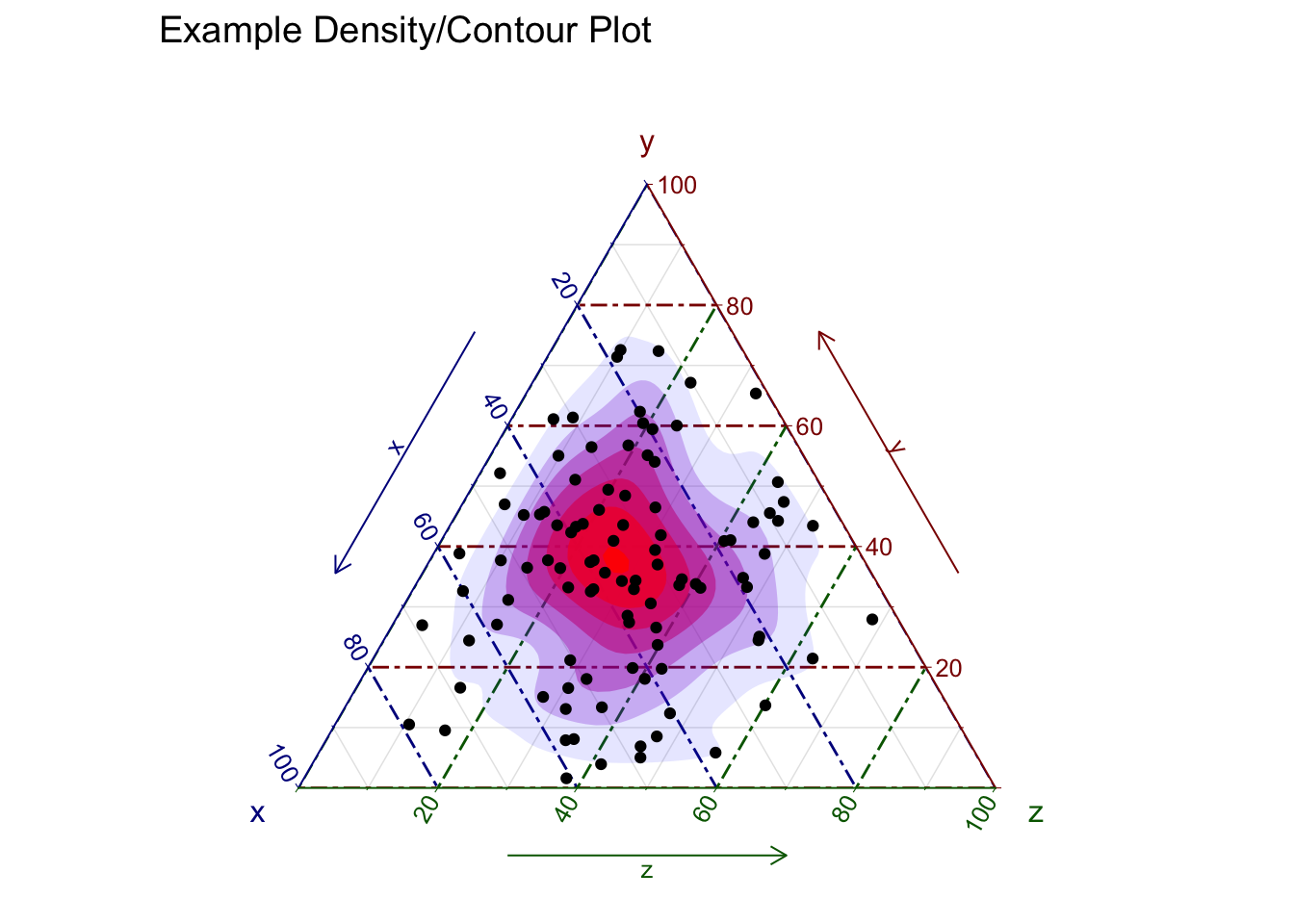ggtern is an extension to ggplot2 specifically for the plotting of ternary diagrams. Ternary diagrams plot three variables and they are commonly used within the fields of chemistry, petrology, mineralogy, metallurgy, materials-science, genetics and game-theory, among others. Whereas a simplex diagram is the general case for $$k$$, in $$k+1$$ dimensions, the ternary diagram is the specific case when $$k=2$$ (producing $$k+1=3$$ vertices).

Given some familiarity with the syntax of ggplot2 it is quite easy to produce publication quality ternary diagrams.

library(ggtern)
set.seed(1)
plot <- ggtern(data = data.frame(x = runif(100),
y = runif(100),
z = runif(100)),
aes(x, y, z))
plot + stat_density_tern(geom = 'polygon',
n         = 200,
aes(fill  = ..level..,
alpha = ..level..)) +
geom_point() +
theme_rgbw() +
labs(title = "Example Density/Contour Plot")    +
scale_fill_gradient(low = "blue",high = "red")  +
guides(color = "none", fill = "none", alpha = "none")The example above is provided by Nicholas Hamilton and published on the webpage of the ggtern package. Check out the webpage for more information and more examples of ternary diagrams using this great library.